求知 文章 文库 Lib 视频 iProcess 课程 认证 咨询 工具 讲座 Modeler Code

 Python教程 Python快速入门 Python概述 Python环境安装 Python基本语法 Python变量类型 Python基本运算符 Python决策 Python循环 Python数据类型 Python字符串 Python列表 Python元组 Python字典 Python日期时间Date/Time Python函数 Python模块 Python文件I/O Python异常处理 高级教程 Python 3开发网络爬虫(一) Python 3开发网络爬虫(二) Python 3(三): 伪装浏览器 Python 3(四): 登录 Python面向对象 Python正则表达式 Python CGI编程
Python基本运算符
 469 次浏览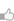47次捐助

Python算术运算符：

[ 查看示例 ]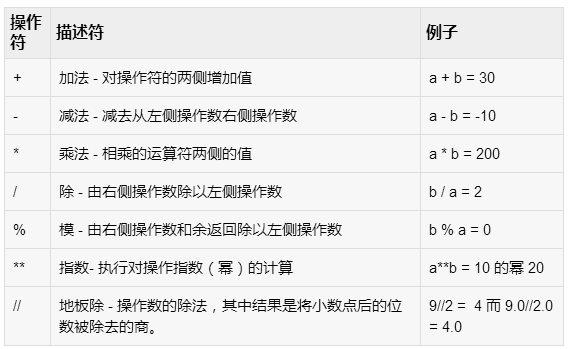Python的比较操作符：

[ 查看示例 ]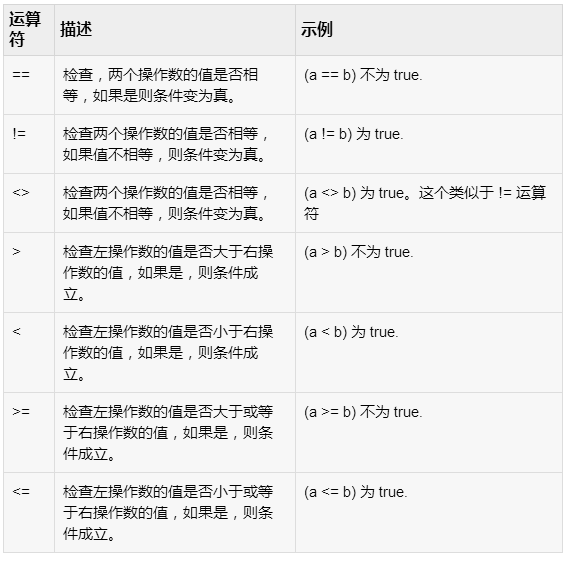Python赋值运算符：

[ 查看示例 ]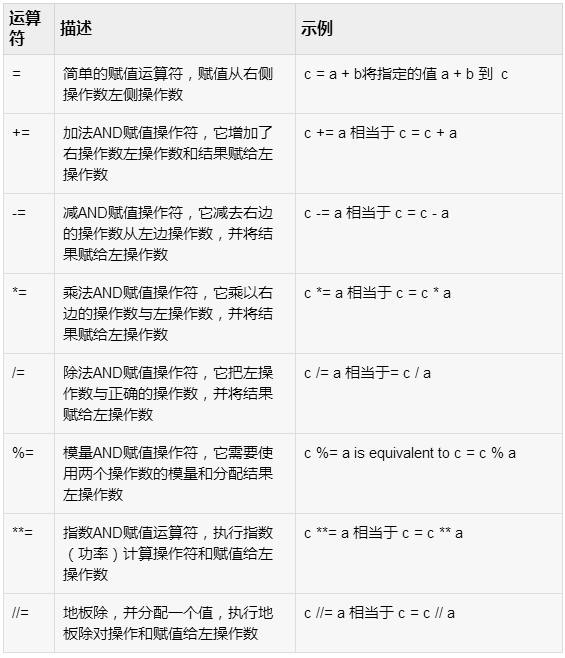Python位运算符：

a = 0011 1100

b = 0000 1101

-----------------

a&b = 0000 1100

a|b = 0011 1101

a^b = 0011 0001

~a = 1100 0011

Python语言支持下位运算符

[ 查看示例 ]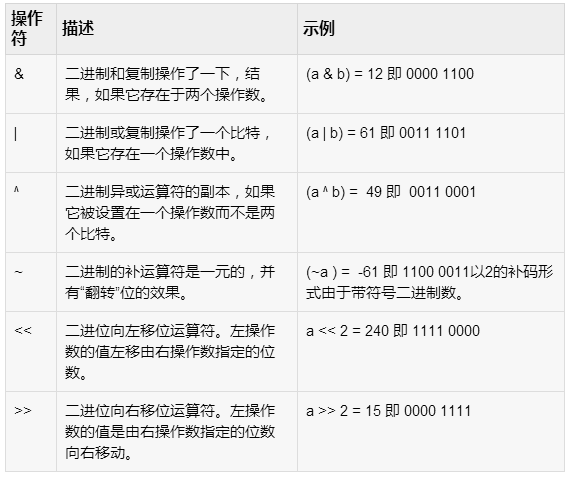Python逻辑运算符：

Python语言支持以下逻辑运算符。假设变量a持有10和变量b持有20则：

[ 查看示例 ]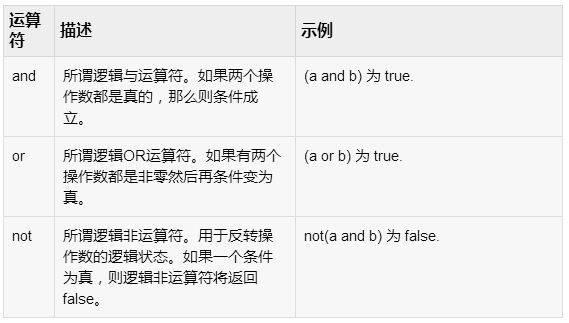Python成员运算符：

[ 查看示例 ]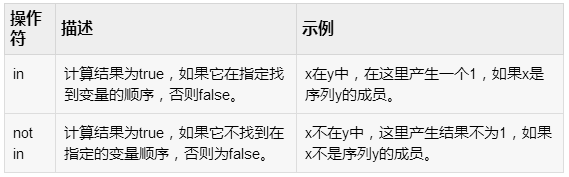Python标识运算符：

[ 查看示例 ]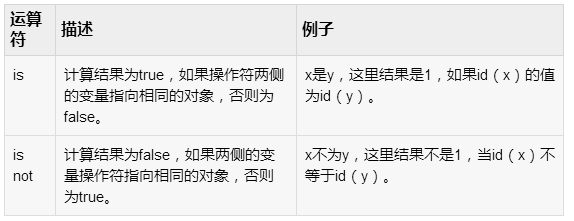Python运算符优先级

[ 查看示例 ]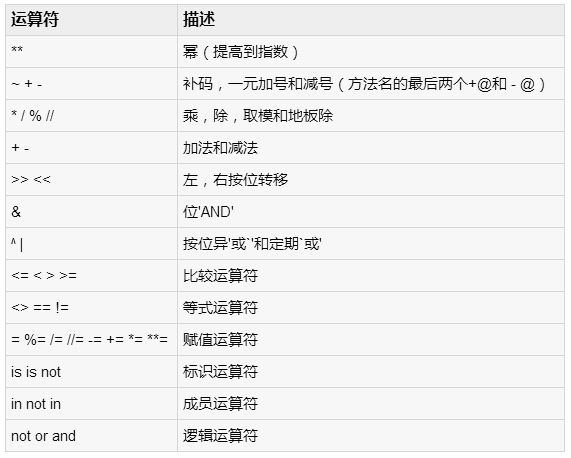您可以捐助，支持我们的公益事业。 1元 10元 50元 认证码：必填
 469 次浏览47次捐助